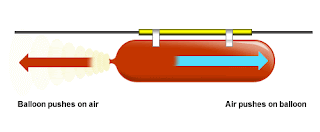## Pages

### 2.2 Dynamics (Newtons laws of motion)

" Sir Isaac Newton (25 December 1642 – 20 March 1726/27) was an English physicist and mathematician (described in his own day as a "natural philosopher") who is widely recognised as one of the most influential scientists of all time and a key figure in the scientific revolution."-wikipedia

In this section we'll discuss Newtons laws of motion.

Newtons laws of motion: Newton's law of motion was first stated in his one of the most famous books he published, Philosophiæ Naturalis Principia Mathematica ("Mathematical Principles of Natural Philosophy"). Just for your curiosity, here's the original text of the Newton's law of motion which was written in latin,

Newton's $1^{st}$ laws of motion: "Every body persists in its state of being at rest or of moving uniformly straight forward, except insofar as it is compelled to change its state by force impressed"

Newton's first law states that when an objects is at rest in any given frame it will continue to remain at rest unless acted upon by a force and and objects moving in a straight line at a given velocity continues its motion in a straight lines unless acted upon by the force to change the direction.

Newton's $2^{nd}$ laws of motion: "The alteration of motion is ever proportional to the motive force impress'd; and is made in the direction of the right line in which that force is impress'd."

$$F=ma$$

Newton's second law simply gives us the definition of the quantity called force. As you can see that in the above figure that the amount of acceleration produced is proportional to the mass of an objects for the same amount of the force impressed. Larger ball has the higher mass so the acceleration is lower than that for the ball with lower mass. Important note: "We can thus say that mass is the property of an objects which resists change in motion"

Newton's $3^{rd}$ laws of motion: "To every action there is always opposed an equal reaction: or the mutual actions of two bodies upon each other are always equal, and directed to contrary parts."courtesy:bbc.co.uk
What this law states is that whatever draws or presses another is as much drawn or pressed by that other. If you press a stone with your finger, the finger is also pressed by the stone. If a horse draws a stone tied to a rope, the horse (if I may so say) will be equally drawn back towards the stone: for the distended rope, by the same endeavour to relax or unbend itself, will draw the horse as much towards the stone, as it does the stone towards the horse, and will obstruct the progress of the one as much as it advances that of the other. If a body impinges upon another, and by its force changes the motion of the other, that body also (because of the equality of the mutual pressure) will undergo an equal change, in its own motion, toward the contrary part. The changes made by these actions are equal, not in the velocities but in the motions of the bodies; that is to say, if the bodies are not hindered by any other impediments. For, as the motions are equally changed, the changes of the velocities made toward contrary parts are reciprocally proportional to the bodies.-wikipedia

EX: A 0.140-kg model rocket accelerates at 45.5 m/s/s on takeoff. Determine the upward force?

Soln: Upward force= weight of the rocket + force on forward motion
=(1.373+6.37)Newton
=7.743N

If you want more information on the Newtons laws of motion,  you can also follow this easy to follow notes on Newton's laws of motion.

references:wikipedia, university physics, nelkon and parker.